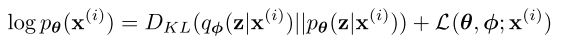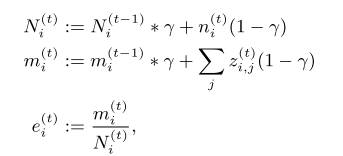# 论文笔记-Discrete Latent Variables Based Generation

• VQ-VAE: Neural Discrete Representation Learning (NIPS2017)
• VQ-VAE2: Generating Diverse High-Resolution Images with VQ-VAE-2
• DALL-E: Zero-Shot Text-to-Image Generation
• VideoGPT: Video Generation using VQ-VAE and Transformers
• LVT: Latent Video Transformer
• Feature Quantization Improves GAN Training (ICML2020)
• DVT-NAT: Fast Decoding in Sequence Models Using Discrete Latent Variables (ICML2018)
• NWT: Towards natural audio-to-video generation with representation learning
• NUWA: Visual Synthesis Pre-training for Neural visUal World creAtion

## VQ-VAE

### Auto-Encoder### VAE• 先验 $p_{\theta}(z)$

• 似然 $p_{\theta}(x|z)$

• 后验 $p_{\theta}(z|x)$这里用到的就是一种在参数技巧。直接生成均值和方差，把后验分布这个转换成一个多维的正态分布。### VQ-VAE

VAE生成的是连续的潜空间，AE生成的是离散的潜空间。那么问题来了，这不是回到原始AE了吗。确实，VQ-VAE本质上更像AE，而不是VAE。但是不同于AE的是，对于vanilla AE，一个训练样本/图像对应一个fixed vector(维度为latent_dims)，也就是潜空间中的一个点。而VQ-VAE则是将图像中的一个patch映射到一个codebook中的一个embedding vector(可视化这些codes可能会有有趣的发现)。可以这么说，一个样本/图像是由潜空间中的多个点构成的，这个潜空间就我们需要学习/维护的codebook。

“Introducing the VQ-VAE model, which is simple, uses discrete latents, does not suffer from “posterior collapse” and has no variance issues. “ 这是论文中的一句话。

Unlike VAEs with continuous latent spaces, VQVAEs use a discrete space by having a codebook containing a large number of continuous vectors. The VQVAE encoding process maps an image to a continuous space then for each spatial location changes each vector to the closest one in the codebook.

Brief recap of posterior collapse in case you’re not sure: my understanding is that VAE models struggle with posterior collapse when (a) the latents contain little information about the input data and (b) a powerful generative model (e.g. an autoregressive decoder) is used that can model the data distribution without any latents. At the start of training the latents often contain little information about the data so the generative model can ignore them and focus on modelling the data distribution on its own. This results in a lack of gradients to the encoder amplifying the problem and so the latents are never used (i.e. posterior collapse).

Specifically with VQVAEs the latents (although discrete) are pretty high dimensional so can store a LOT of information about the input, so this helps with (a). As for (b), the decoder tends to be a fairly simple conv net so the latents are definitely needed to reconstruct the input.

As for the variance issues since VAE encoders are probabilistic, training requires sampling the encoder outputs which can have high variance. Gaussian VAEs bypass this using the reparameterisation trick (here is a great discussion on this https://ermongroup.github.io/cs228-notes/extras/vae/). While VQVAEs have deterministic encoders, the discretisation process can introduce variance, however, they use the straight through estimator which is biased but has low variance, I believe. This leads to other issues such as codebook collapse, where some codes are never used. DALL-E on the other hand uses Gumbel Softmax.• 52-65行计算 l2 距离，然后选择距离最小的index，再通过这个index去codebook中选取对应的vector

• 这里的 quantized_latents 也就是我们想到得到的 $z_q$

• 68-69行代码对应下式中后两个lossEMA update codebook：• $e_{top}\leftarrow Quantize(h_{top})$ 中 $h_{top}$ 就是encoder的输出，通过量化(映射)之后得到 $e_{top}$
• 对于bottom也是一样的

prior training:

• $T_{top}$ 就是 $e_{top}$ 的序列

• $p_{top}=TrainPixelCNN(T_{top})$ 训练自回归模型

## VideoGPT• VQ-VAE training
• VideoGPT training## DVT-NAT

Fast Decoding in Sequence Models Using Discrete Latent Variables, (ICML2018)

#### Abstract

• 提出了一种对目标序列进行离散建模的方法，能有效提高自回归建模的效率，从而提升解码速度

To overcome this limitation, we propose to introduce a sequence of discrete latent variables $l_1 . . . l_m$, with $m < n$, that summarizes the relevant information from the sequence $y_1 . . . y_n$. We will still generate $l_1 . . . l_m$ autoregressively, but it will be much faster as $m < n$ (in our experiments we mostly use $m = n/8$ ). Then, we reconstruct each position in the sequence $y_1 . . . y_n$ from $l_1 . . . l_m$ in parallel.

以前的seq2seq方式是 $(x_1,…,x_L) \rightarrow (y_1,…,y_n)$ ，现在是 $(x_1,…,x_L) \rightarrow (l_1,..,l_m)\rightarrow (y_1,…,y_n)$ ,其中 $l_1,…,l_m$ 的生成依然是自回归的，但是长度更短， 所以提高了效率。

• 将 latent transformer 应用在机器翻译上，提升翻译效率。但是在BLEU上仍然比自回归的方法要差很多。

### Contribution

• 提出了一个基于离散潜变量的快速解码框架
• 提出新的离散化技术，能有效缓解VQ-VAE中的index collapse问题
• latent transofmer 应用在机器翻译上，提升了翻译速度

#### Discretization Techniques

• Gumbel-Softmax

• 将argmax/argmin可微化。将采样过程用可导的softmax代替，同时加上gumbel noise使得最终的结果跟
• PyTorch 32.Gumbel-Softmax Trick - 科技猛兽的文章 - 知乎 https://zhuanlan.zhihu.com/p/166632315• Improved Semantic Hashing

• Vector Quantization

#### Decomposed Vector Quantization

##### motivation

index collapse, where only a few of the embedding vectors get trained due to a rich getting richer phenomena##### Sliced Vector Quantization
• break up the encoder output enc(y) into n_d smaller slices, like multi-head attention in transformer (eq. 11)#### Latent Transformer

• Main Steps

• VAE encoder encodes target sentence $y$ into shorter discrete latent variables $l$ (parallel)
• Latent prediction model - Transformer, is trained to predict $l$ from source sentence $x$ (autoregressive)
• VAE decoder decodes predicted $l$ back to sequence $y$ (parallel)
• Loss Function

• reconstruction loss $l_r$ from VAE
• latent prediction loss $l_{lp}$ from Latent Transformer
• in first 10k steps, true targets $y$ is given to transformer-decoder instead of decompressed latents $l$ which ensures self-attention part has reasonable gradients to train the whole architecture
• Architectures of VAE

• Encoder

• conv residual blocks + attention + conv to scale down the dimension
• $C = n/m, C=2^c$, in the setting, $C=8, c=3$• Decoder

• conv residual blocks + attention + up-conv to scale up the dimension
• Transformer DecoderXie Pan

2021-09-12

2021-12-08